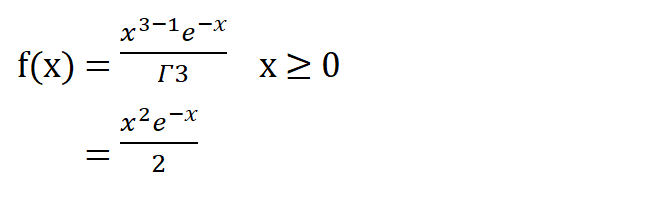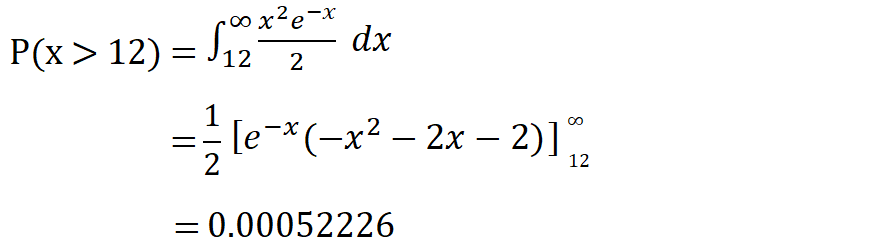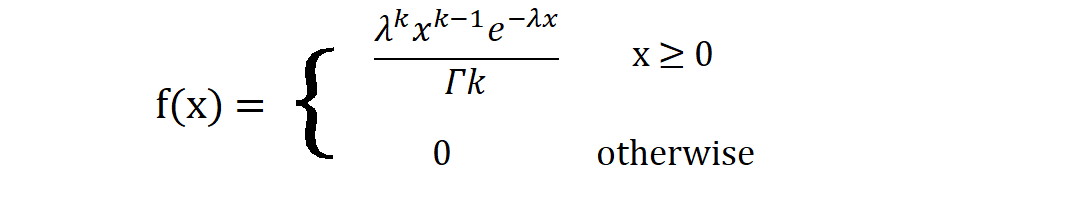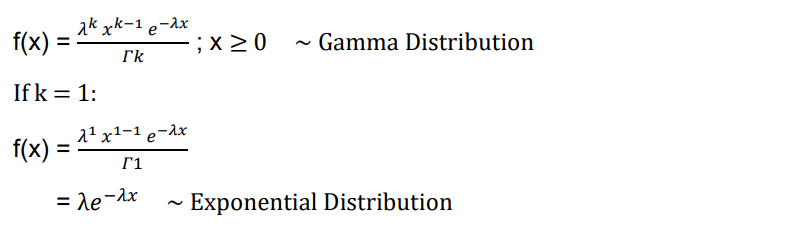## Introduction

Whenever we want to know the probability for the waiting time until the k-th number of success occurs for a particular event, while the mean rate of success per unit time is known, then we can use Gamma Distribution to find out the solution. In probability and statistics, Gamma Distribution falls under a family of continuous probability distributions.Here:

• x = random variable
• k = mean

Therefore, the following are the properties of Gamma Distribution:

• Mean = k
• Variance = k

## Gamma Distribution

Let us consider an example of a city, where the daily consumption of electric power in millions of kilowatt-hours can be treated as a random variable having Gamma Distribution with k = 3. Then the Gamma Distribution of this scenario will be:If the powerplant of this city has a daily capacity of 12 million kilowatt-hours, then the probability that the power supply will be inadequate for a given day is:We can infer that the answer is correct as city power stations leave very little margin of probability for power failure or inadequacy.#### Special Cases of Gamma Distribution

Some of the special cases of Gamma Distribution are:

• Erlang Distribution

In this case, the Gamma Distribution formula is generalized. Usually, when we consider Gamma Distribution, we assume the value of λ to be 1. This is not assumed in Erlang Distribution. Therefore, Erlang Distribution is also called as General Gamma Distribution.• Chi-squared Distribution

In this case, the variance is twice as much as the mean, unlike that of Gamma Distribution. Chi-squared Distribution becomes the basis of the Chi-square Test in the Hypothesis Testing concept. The Gamma formula changes to:Particular case:

• Exponential Distribution

When we want to find the probability for the waiting time 1st ever successful occurrence of the event, then the distribution follows Exponential Distribution.#### Implementation

Let us try to code the example that we have solved earlier. First, we define a function that finds out the factorial of a number. Then, we create a function that can compute the value of gamma distribution and finally we pass the values to yield the answer.

				
fun factorial(n: Double): Double
{
var x = 1.0
var i = 2.0
while (i &lt;= n)
{
x *= i
i++
}
return x
}


				
fun gamma(x: Double, k: Double): Double
{
return Math.exp(-x) * Math.pow(x, k-1) / factorial(k-1)
}



Note: Since the gamma function has been interpreted as the factorial of 1 less than the number, we have given that notation to the code. However, this code will work aptly as long as the variable k is an integer.

				
var k = 3.0
var x = 12.0
if (x &lt; 0)
{
println(&quot;0&quot;)
}
else
{
println(gamma(x, k))
}



Output:

				
4.423832894396311E-4